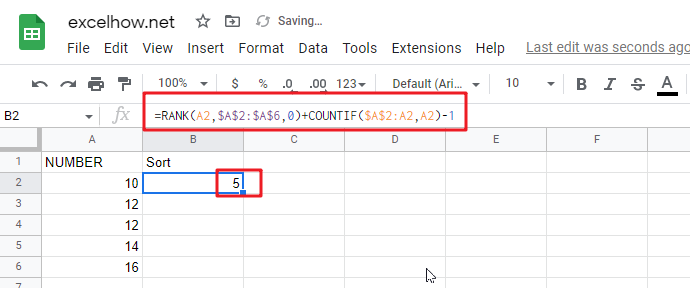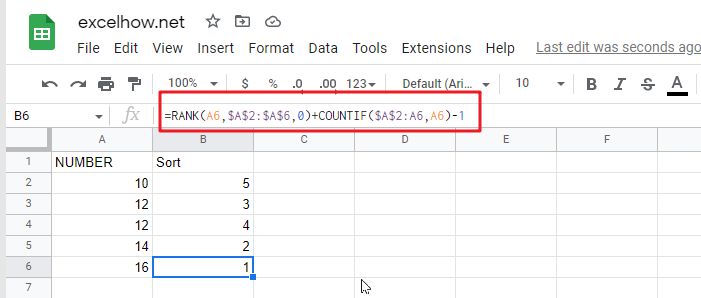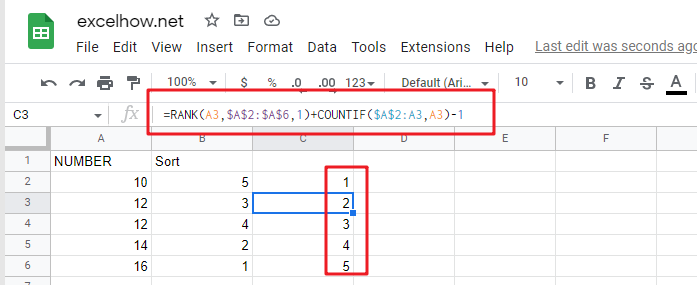# Rank Numbers without Repetitive Ranks in google sheets

This article will guide you how to rank numbers in a range with unique ranking in google sheets. How to use the Rank function in google sheets to get a unique ranking for ranges with duplicate values.

## Ranking without Repetitive Ranks

Suppose you try to use the RANK function to rank values in a range of cells that have two or more duplicate values. And the RANK function will return the same ranking for duplicate values in the cell range.

For example, if you have a list of data containing values in column A (10, 12, 12, 14, 16), the RANK function will return a list of ranked values in another column as `5, 4, 4, 2, 1`. The returned result may not be the one that you expect. Because you maybe expect the result as` 5, 4, 3, 2, 1`. At this time you can use the RANK function and in combination with COUNTIF function to build a new complex google sheet formula to return a unique ranking value.

The formula is as follows：

`=RANK(A2,\$A\$2:\$A\$6,0)+COUNTIF(\$A\$2:A2,A2)-1`

You can enter the above google sheets formula in cell B2, and then press the Enter key to make the formula take effect;Fill Handle can be dragged down until the cell B6, so that other cells can also apply the formula.From the above screenshot you can see that the unique ranking results are sorted in descending order.

If you wish the unique ranking results for the given cell range is sorted in ascending order, you can change the order type of the RANK function to `1`, the formula is changed as follows:

`=RANK(A2,\$A\$2:\$A\$6,1)+COUNTIF(\$A\$2:A2,A2)-1`

You can enter the above google sheets formula in cell C2, and then press the Enter key to make the formula take effect;

Then you can be dragged Fill Handle can be dragged down until the cell C6, so that other cells can also apply the formula.